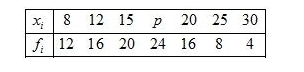# Find the value of p for the following distribution whose mean is 16.6.

Question:

Find the value of p for the following distribution whose mean is 16.6.Solution:

Given:Mean 16.6

First of all prepare the frequency table in such a way that its first column consist of the values of the variate $\left(x_{i}\right)$ and the second column the corresponding frequencies $\left(f_{i}\right)$.

Thereafter multiply the frequency of each row with corresponding values of variable to obtain third column containing $\left(f_{i} x_{i}\right)$.

Then, sum of all entries in the column second and denoted by $\sum f_{i}$ and in the third column to obtain $\sum f_{i} x_{i}$.We know that mean, $\bar{X}=\frac{\sum f_{i} x_{i}}{\sum f_{i}}$

$16.6=\frac{1228+24 p}{100}$

By using cross multiplication method

$1228+24 p=16.6 \times 100$

$24 p=1660-1228$

$=\frac{432}{24}$

$=18$

Hence, $p=18$